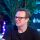# How to Get Unique Values From an Array or Collection

#### Apr 23, 2021### tldr; Use Sets and spread results

#### From an array

``````const unique = [...new Set(["one", "two", "one"])]
// ["one", "two"]``````

#### From a collection

``````const unique = [...new Set([{
value: "one",
},{
value: "two",
},{
value: "one",
}].map((obj) => obj.value))]
// ["one", "two"]``````

### Why does this work?

Let's break down what we're doing here.

#### What is a Set?

"The Set object lets you store unique values of any type, whether primitive values or object references."

That's really it. By definition a JavaScript set is a collection of values that may only exist once. As of ES2015, this comparison is done by the same algorithm as "===".

``[...set]``
`const unique = [...new Set(["one", "two", "one"])]`